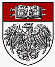#University of Chicago Mathematics Department Inquiry-Based Learning (IBL) Scripts

Webpage maintained by: John Boller, Senior Lecturer in Mathematics

### Basic Description

Inquiry-Based Learning is a teaching technique whereby mathematics students are presented with scripts of definitions, axioms, and theorems, and are expected to prove the results for themselves. They present the proofs in class, and their fellow students criticize the proofs under the supervision of the instructor.

### History at Chicago

Modern IBL methods have been used in the Mathematics Department since 2004. Starting with one section of Honors Calculus, the department has since expanded IBL to include courses in Real Analysis, Honors Calculus, Elementary Number Theory, and Elementary Geometry.

### Honors Calculus I, II, III (Math 16100-16200-16300)

#### Materials

Here is a proposed Calendar for the Autumn Quarter.
Here is the LaTeX Assignment and the corresponding Template.tex file.
Here is the assignment Fun with Sets in (.pdf) and (.tex) formats.

#### Scripts

Script 0: The Natural Numbers and Mathematical Induction in (.pdf) and (.tex) formats.
Script 1: Sets, Functions, and Cardinality (.pdf) and (.tex) formats.
Script 2: Introducing the Continuum (.pdf) and (.tex) formats.
Script 3: The Topology of the Continuum in (.pdf) and (.tex) formats.
Script 4: Connectedness, Boundedness, and Compactness in (.pdf) and (.tex) formats.
Script 5: Continuous Functions in (.pdf) and (.tex) formats.
Script 6: The Field Axioms in (.pdf) and (.tex) formats.
Script 7: The Rational Numbers in (.pdf) and (.tex) formats.
Script 8: The Real Numbers in (.pdf) and (.tex) formats.
Script 9: Sequences and Limits in (.pdf) and (.tex) formats.
Script 10: Limits and Derivatives of Functions in (.pdf) and (.tex) formats.
Script 11: Uniform Continuity and Integration in (.pdf) and (.tex) formats.
Script 12: The Fundamental Theorem of Calculus and the Inverse Function Theorem in (.pdf) and (.tex) formats.
Script 13: Series in (.pdf) and (.tex) formats.